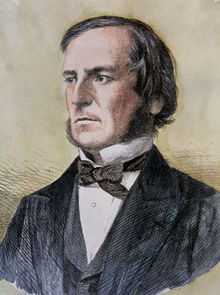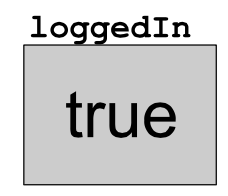# Booleans

How do we write code that tells us whether a user is logged in to our program? Booleans are the solution to these questions.

### What are Booleans?

A boolean refers to a value that is true or false. Those are the only values of a boolean expression, and these are useful if we want to check if something is true or false.

### Meet George Boole

Let's meet the fellow behind the name, "booleans," George Boole. Mr. Boole was an English-born mathematician, philosopher, and logician. He introduced boolean algebra in The Mathematical Analysis of Logic (1847) and Investigation of the Laws of Thought (1854). Many consider him to be one of the founders of Computer Science.### Working with Booleans

How about an example? Let's create a variable and set it equal to true. Then, we'll print the variable.

We first want to declare the variable, `loggedIn`, and set it to true or false.

``````boolean loggedIn = false;
``````

We can similarly set the variable to true instead:

``````boolean loggedIn = true;
``````

You can imagine a boolean variable as a box that can hold only the values true or false. The box below shows the current value of `loggedIn`:Notice that we do not need to have quotations around `true` or `false`.

The full example looks like:

``````// In this program we declare a boolean
// value to represent whether the user
// is logged in, and then print it out.
public class LoggedIn extends ConsoleProgram
{
public void run()
{
boolean loggedIn = false;
System.out.println("User logged in?: " + loggedIn);
}
}
``````

Remember, use booleans if you want to set a variable to true or false!